## Model Coverage Reports for MATLAB Functions

After collecting coverage for your model, you can generate a coverage report that summarizes the coverage results and the details for each block. If your model contains MATLAB Function blocks, you can view the coverage results line-by-line for the MATLAB® code inside the block. The coverage analysis looks slightly different for each type of MATLAB function:

### Coverage Reports for MATLAB Functions in a MATLAB Function Block

Consider the model coverage report for the MATLAB function `run_intersect_test`, which is defined inside a MATLAB Function block.

Below the linked function name is a link to the section of the report for the parent MATLAB Function block that contains the code for the `run_intersect_test` function.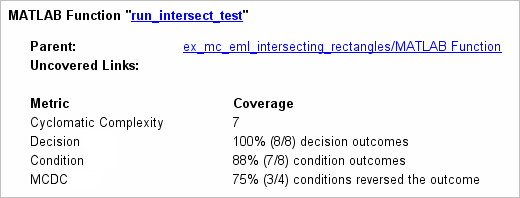The top half of the report for the function summarizes its model coverage results. The coverage metrics for `run_intersect_test` include decision, condition, and MCDC coverage. You can understand these metrics by examining the code for `run_intersect_test`.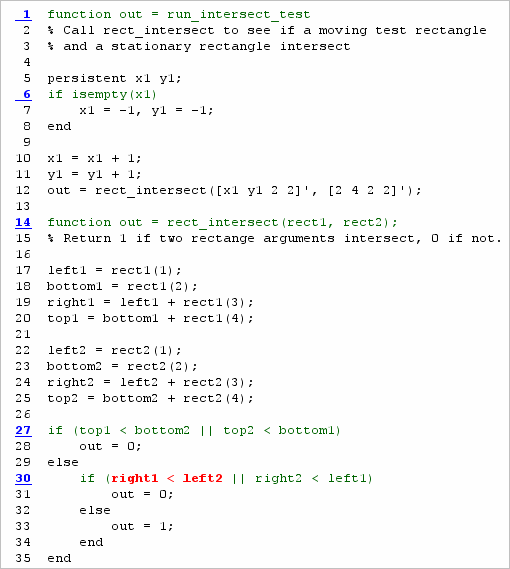Lines with coverage elements are marked by a highlighted line number:

• Line 1 receives decision coverage that indicates whether the top-level function `run_intersect_test` executed.

• Line 6 receives decision coverage for the `if` statement.

• Line 14 receives decision coverage that indicates whether the local function `rect_intersect` executed.

• Lines 27 and 30 receive decision, condition, and MCDC coverage for the `if` statements and conditions.

The condition `right1 < left2` in line 30 displays in red, which indicates that this condition did not evaluate all of its possible outcomes. The coverage report displays which of the outcomes remains unsatisfied by the coverage analysis.

The coverage report includes detailed information for each of these lines of code. Click the links to open the editor to the associated line in the report.

#### Coverage Summary

The Coverage Details pane displays the metrics that summarize coverage for the entire `run_intersect_test` function.The conclusions from the coverage summary are:

• There are eight decision outcomes reported for `run_intersect_test` in the line reports:

• One for line 1 (executed)

• Two for line 6 (`true` and `false`)

• One for line 14 (executed)

• Two for line 27 (`true` and `false`)

• Two for line 30 (`true` and `false`).

The decision coverage for each line shows 100% decision coverage. This result means that decision coverage for `run_intersect_test` is eight of eight possible outcomes, or 100%.

• There are four conditions reported for `run_intersect_test` in the line reports. Lines 27 and 30 each have two conditions, and each condition has two condition outcomes (`true` and `false`), for a total of eight condition outcomes in `run_intersect_test`. All conditions tested positive for both the `true` and `false` outcomes except the first condition of line 30 (```right1 < left2```). This means that condition coverage for `run_intersect_test` is seven of eight, or 88%.

• The MCDC coverage tables for decision lines 27 and 30 each list two cases of decision reversal for each condition, for a total of four possible reversals. Only the decision reversal for a change in the evaluation of the condition `right1 < left2` of line 30 from `true` to `false` did not occur during simulation. This means that three of four, or 75% of the possible reversal cases were tested for during simulation, for a coverage of 75%.

#### Coverage for Line 1

The first line of every MATLAB function configured for code generation receives coverage analysis as a decision. The decision indicates that the function executed as a response to being called.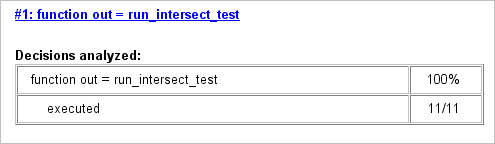The coverage report for `run_intersect_test` displays 100% decision coverage, which indicates that the function executed at least once. The decision table additionally shows that the function executed eleven times.

#### Coverage for Line 6

The Decisions analyzed table indicates that the decision in line 6, `if isempty(x1)`, executed a total of eleven times. The decision evaluated to `true` for one time step and false for ten time steps. Because both possible outcomes occurred, decision coverage is 100%.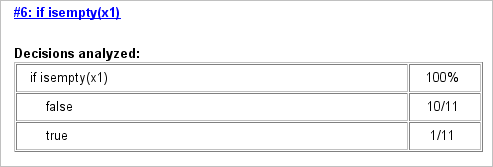#### Coverage for Line 14

The Decisions analyzed table indicates that the local function `rect_intersect` executed during testing, and consequently received 100% coverage.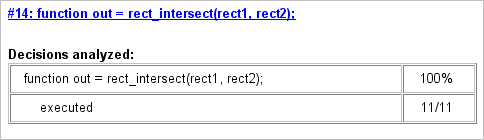#### Coverage for Line 27

The Decisions analyzed table indicates that there are two possible outcomes for the decision in line 27, `true` and `false`. Five of the eleven times the expression executed, the decision evaluated to `false`. The remaining six times, the decision evaluated to `true`. Because both possible outcomes occurred, decision coverage is 100%.

Additionally, the Conditions analyzed table shows that, because this decision consists of two conditions linked by a logical OR (`||`) operation, only one condition must evaluate `true` for the decision outcome to be `true`. If the first condition evaluates to `true`, there is no need to evaluate the second condition. This is called logical short circuiting. The first condition, ```top1 < bottom2```, was evaluated eleven times and was `true` twice. This result means that the second condition, `top2 < bottom1` was evaluated only nine times. The condition evaluated to `true` four times, which brings the total `true` occurrences for the decision to six, which matches the number in the Decisions analyzed table.

MCDC coverage looks for decision reversals that occur because one condition outcome changes from `T` to `F` or from `F` to `T`. The MC/DC analysis table identifies possible combinations of outcomes for the conditions that lead to a reversal in the decision. The character `x` indicates a condition outcome that is irrelevant due to logical short circuiting. Condition outcome combinations that are not achieved during simulation are marked with a set of parentheses. For line 27, because each condition independently affects the decision outcome, the reported MCDC coverage is 100% and the MC/DC analysis table does not include parentheses around any condition outcome combinations.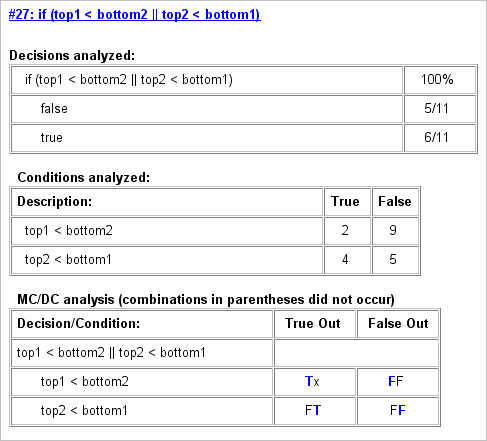#### Coverage for Line 30

The line 30 decision, ```if (right1 < left2 || right2 < left1)```, is nested in the `else` case of the `if` statement on line 27. Therefore, the line 30 decision is evaluated only if the line 27 decision is `false`. Because the line 27 decision evaluated `false` five times, line 30 is evaluated five times, three of which are `false`. Because both the `true` and `false` outcomes are achieved, decision coverage for line 30 is 100%.

Because line 30, like line 27, has two conditions related by a logical OR operator (`||`), condition 2 is evaluated only if condition 1 is `false`. Because condition 1 evaluates `false` five times, condition 2 is evaluated five times. Of these, condition 2 evaluates `true` two times and `false` three times, which accounts for the two occurrences of the `true` outcome for this decision.

Because the first condition of the line 30 decision does not evaluate `true`, both outcomes do not occur for that condition and the report highlights the condition coverage for the first condition with a rose color. The report also highlights the MCDC coverage in the same way for a decision reversal based on the `true` outcome for that condition.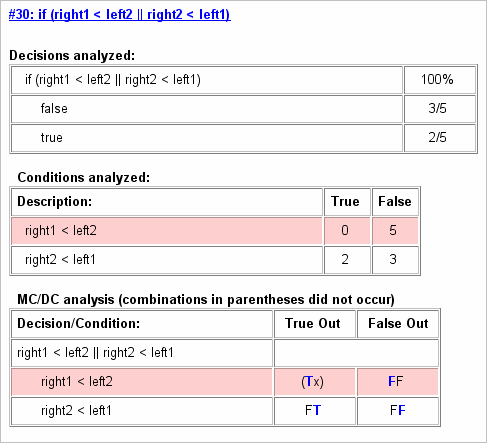### Coverage Reports for Simulink Design VerifierMATLAB Functions

If you configure your MATLAB code for code generation, and the code includes these Simulink® Design Verifier™ functions, you can measure coverage:

For this example, consider a model that contains a MATLAB Function block.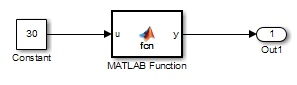The MATLAB Function block contains this code:

```function y = fcn(u) % This block supports MATLAB for code generation. sldv.condition(u > -30) sldv.test(u == 30) y = 1;```

To collect coverage for Simulink Design Verifier MATLAB functions, in the Configuration Parameters dialog box, on the Coverage pane, under Other metrics, select Objectives and Constraints.

After simulation, the model coverage report displays coverage for the `sldv.condition` and `sldv.test` functions. For `sldv.condition`, the expression `u > -30` evaluated to `true` 51 times. For `sldv.test`, the expression `u == 30` evaluated to `true` 51 times.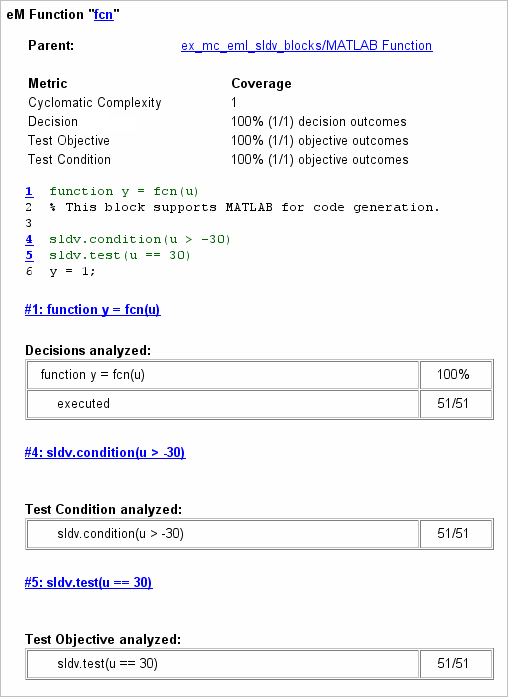For an example of model coverage data for Simulink Design Verifier blocks, see Objectives and Constraints Coverage.

### Coverage Reports for MATLAB Functions in an External File

Using the same model in Model Coverage Reports for MATLAB Functions, suppose the MATLAB functions `run_intersect_test` and `rect_intersect` are stored in an external MATLAB file named `run_intersect_test.m`.

To collect coverage for MATLAB functions in an external file, in the Configuration Parameters dialog box, on the Coverage pane, select Coverage for MATLAB files.

After simulation, the model coverage report summary contains sections for the top-level model and for the external function.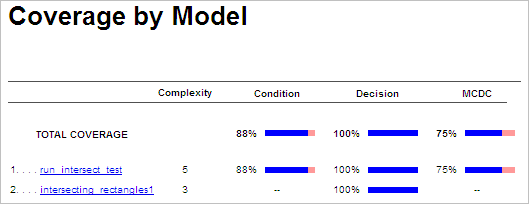The model coverage report for `run_intersect_test.m` reports the same coverage data as if the functions were stored in the MATLAB Function block.

For a detailed example of a model coverage report for a MATLAB function in an external file, see External MATLAB File Coverage Report.